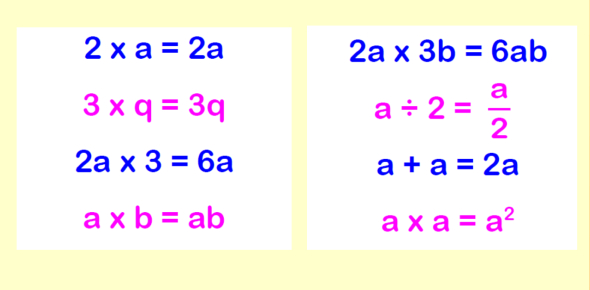# Simplifying Algebraic Expressions Quiz For Grade 7

10 Questions | Attempts: 20977SettingsAre you a student in grade 7th? How easily can you solve algebraic expressions with accuracy? Take this 'Simplifying algebraic expressions quiz' and check your logical and reasoning skills regarding it. An algebraic expression is made up of variables and consonants along with algebraic operations (addition, subtraction, multiplication, etc. ) In this quiz, you need to combine like terms and use the order of operations to simplify algebraic expressions. Do you think you can do so? Go ahead, then!

• 1.
3x + 1 + 8x + 9
• A.

12x + 10

• B.

5x + 8

• C.

11x + 11

• D.

11x + 10

• 2.
2x + 5y - 7x + 8y
• A.

9x + 13y

• B.

5x + 3y

• C.

-5x + 3y

• D.

-5x + 13y

• 3.
4(x + 8) - 9
• A.

4x + 32

• B.

4x + 23

• C.

12x - 9

• D.

4x + 24

• 4.
-3(x + y) + 5(x - y)
• A.

2x - 8y

• B.

-2x + 2y

• C.

8x - 8y

• D.

2x - 8y

• 5.
A + b = b + a is an example of the property called
• A.

• B.

• C.

Both A and B

• D.

None of these

• 6.
What number is known as the multiplicative identity?
• A.

1

• B.

2

• C.

-1

• D.

0

• 7.
3x - 5x
• A.

2x

• B.

-2x

• C.

3x

• D.

8x

• 8.
3x + 2x -3x
• A.

-2x

• B.

2x

• C.

-3x

• D.

3x

• 9.
3w - m + w + 6m
• A.

3w + 5m

• B.

3w + 7m

• C.

4w + 7m

• D.

4w + 5m

• 10.
Ab(c) = (ab)c is an example of what property?
• A.

• B.

Associative Property of Multiplication

• C.

Cumulative Property of Multiplication

• D.

None of these

## Related TopicsBack to top
×

Wait!
Here's an interesting quiz for you.